×#### Thank you for registering.

One of our academic counsellors will contact you within 1 working day.

Click to Chat

1800-1023-196

+91-120-4616500

CART 0

• 0

MY CART (5)

Use Coupon: CART20 and get 20% off on all online Study Material

ITEM
DETAILS
MRP
DISCOUNT
FINAL PRICE
Total Price: Rs.

There are no items in this cart.
Continue Shopping• Complete JEE Main/Advanced Course and Test Series
• OFFERED PRICE: Rs. 15,900
• View Details

```Chapter 5: Operations on Rational Numbers Exercise – 5.2

Question: 1

Subtract the first rational number from the second in each of the following: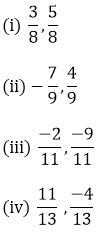Solution: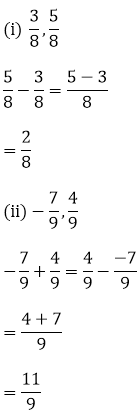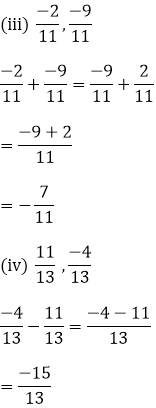Question: 2

Evaluate each of the following: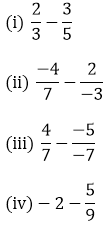Solution: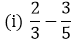LCM of 3 and 5 is 15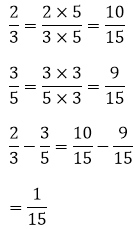LCM of 3 and 7 is 21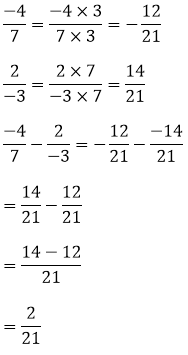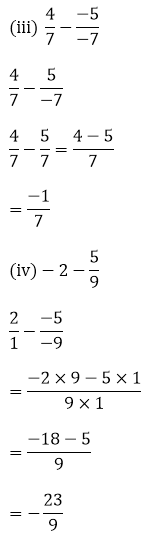Question: 3

The sum of the two numbers is 5/9. If one of the numbers is 1/3, find the other.

Solution:LCM of 3 and 9 is 9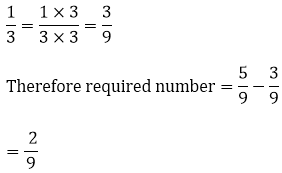Question: 4

The sum of two numbers is -1/3. If one of the numbers is -12/3, find the other.

Solution:

Let the required number be x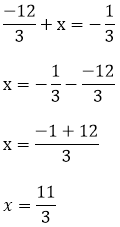The required number is 11/3

Question: 5

The sum of two numbers is – 4/3. If one of the numbers is -5, find the other.

Solution:

Let the required number be x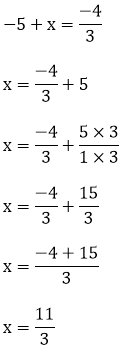The required number is 11/3

Question: 6

The sum of two rational numbers is - 8. If one of the numbers is – (15/7), find the other.

Solution:

Let the required number be xThe required number is – (41/7)

Question: 7

What should be added to – (7/8) so as to get 5/9?

Solution:

Let the required number be xThe required number is 103/72

Question: 8

What number should be added to (-5)/11 so as to get 26/33?

Solution:

Let the required number be x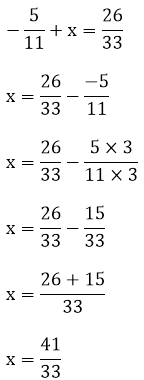The required number is 41/33

Question: 9

What number should be added to (-5)/7 to get (-2)/3?

Solution:

Let the required number be x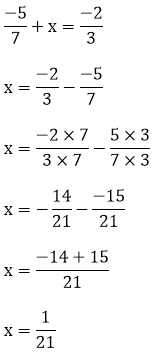The required number is 1/21

Question: 10

What number should be subtracted from -5/3 to get 5/6?

Solution:

Let the required number be x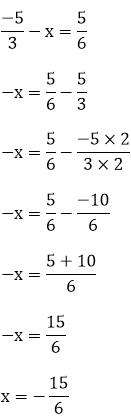The required number is 15/6

Question: 11

What number should be subtracted from 3/7 to get 5/4?

Solution:

Let the required number be x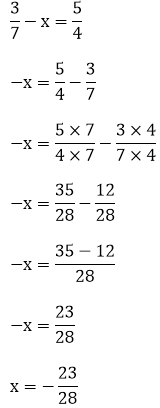The required number is 23/28

Question: 12Solution:

Let the required number be xThe required number is (-7)/5

Question: 13Solution:

Let the required number be xThe required number is 59/30

Question: 14Solution:

Let the required number be xx = 1/4

The required number is ¼

Question: 15

Simplify: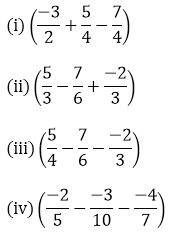Solution: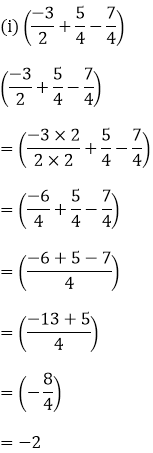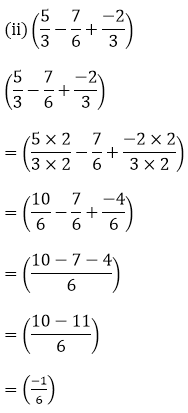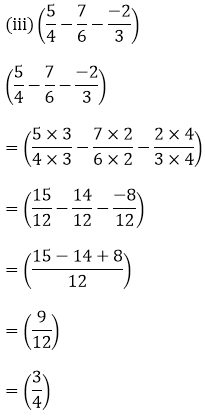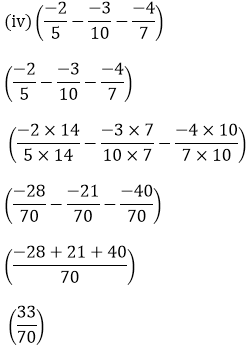Question: 16

Fill in the blanks:Solution: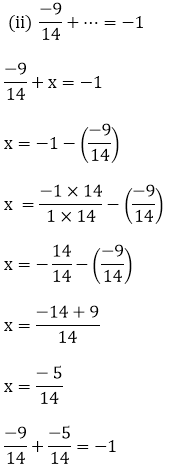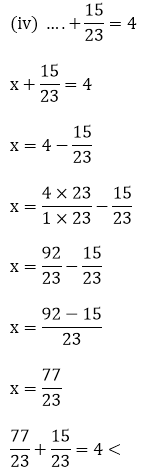```### Course Features

• 728 Video Lectures
• Revision Notes
• Previous Year Papers
• Mind Map
• Study Planner
• NCERT Solutions
• Discussion Forum
• Test paper with Video Solution